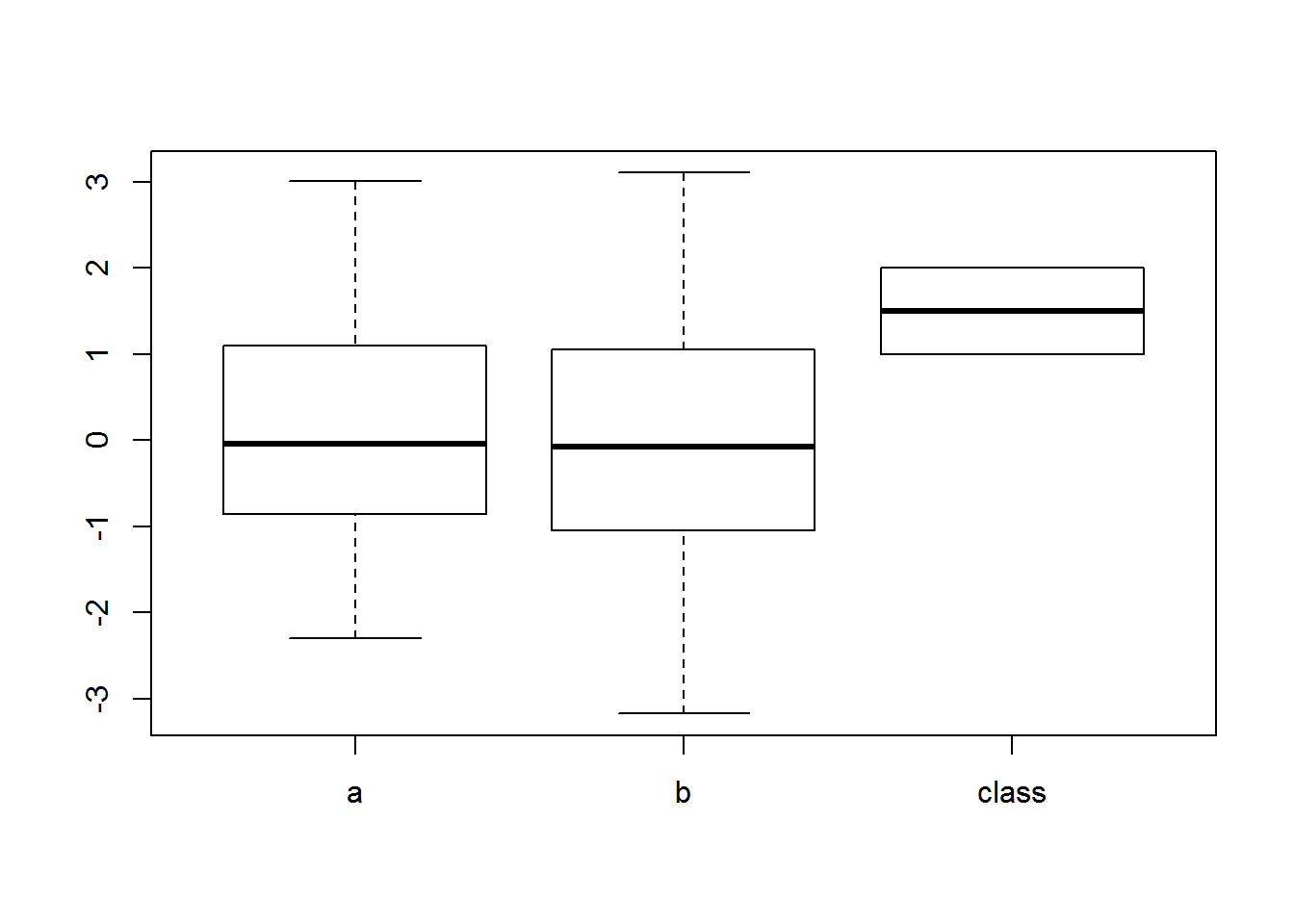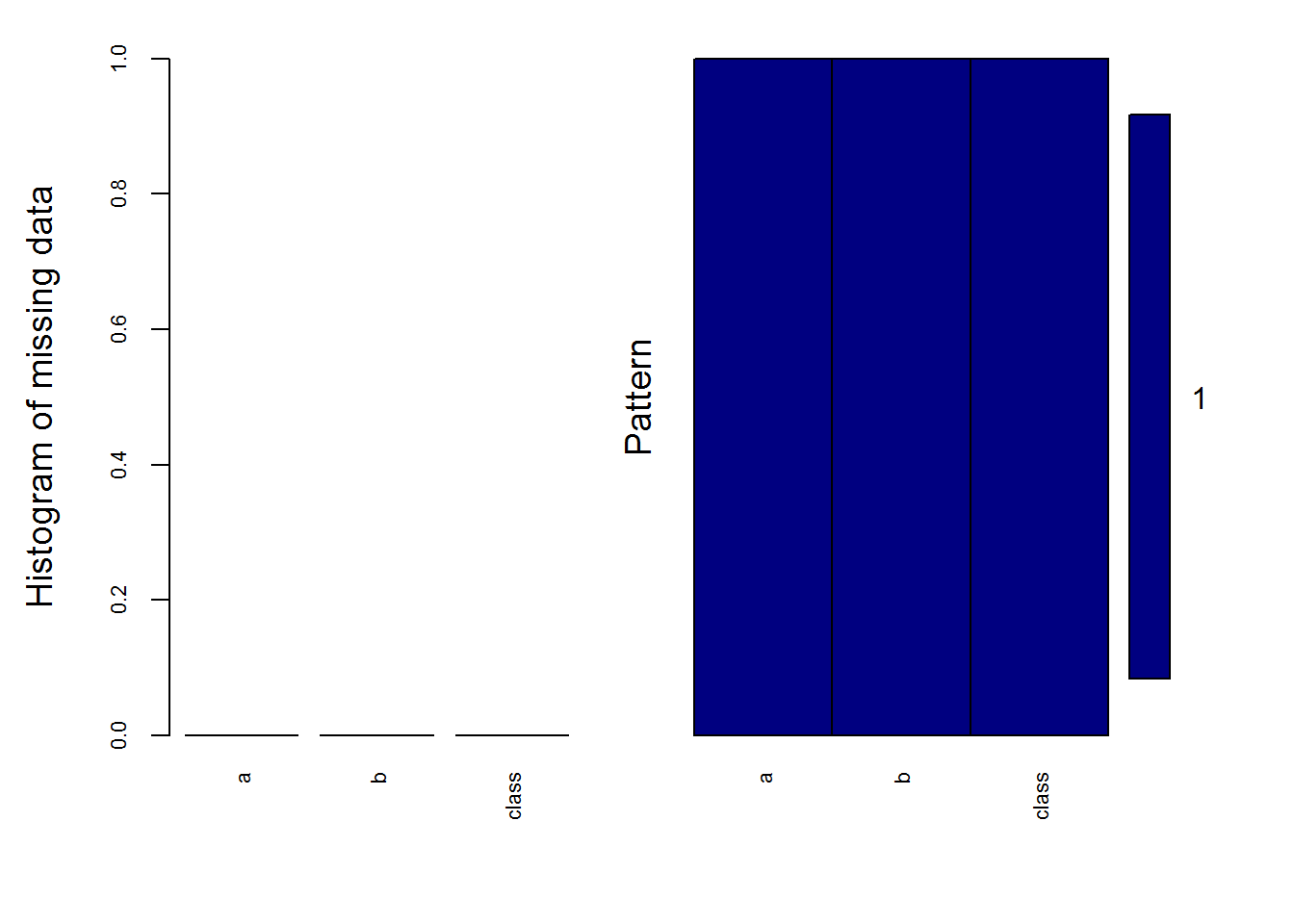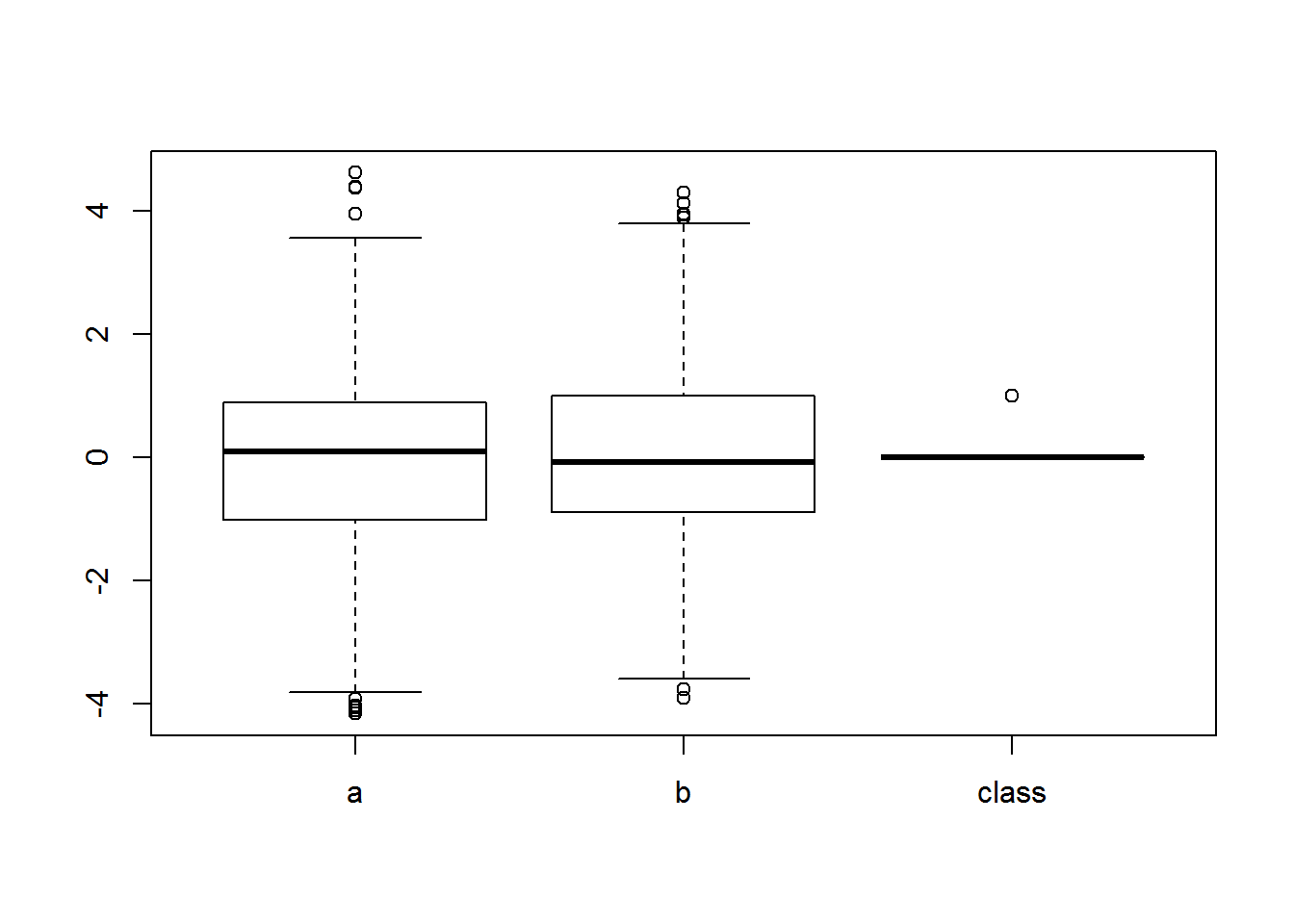There are 100 observation with 3 columns.

library(VIM)
## Loading required package: colorspace
## Loading required package: grid
## Loading required package: data.table
## VIM is ready to use.
##  Since version 4.0.0 the GUI is in its own package VIMGUI.
##
##           Please use the package to use the new (and old) GUI.
## Suggestions and bug-reports can be submitted at: https://github.com/alexkowa/VIM/issues
##
## Attaching package: 'VIM'
## The following object is masked from 'package:datasets':
##
##     sleep
df <- read.csv("C:\\Users\\Charls\\Documents\\CunyMSDS\\Data622\\Assignments\\HW1\\Qn2\\junk1.txt", header = T, sep = ' ')
dim(df)
##  100   3

## EDA for dataset ’junk1.txt"

The given dataset is balanced(i.e both classes are evenly distributed.) a is a numeric value ranges from -2.2 to 3 b is a numeric value ranges from -3.1 to 3 class - 1, 2

Note: Need to know the metadata for this dataset

table(df$class) ## ## 1 2 ## 50 50 summary(df) ## a b class ## Min. :-2.29854 Min. :-3.17174 Min. :1.0 ## 1st Qu.:-0.85014 1st Qu.:-1.04712 1st Qu.:1.0 ## Median :-0.04754 Median :-0.07456 Median :1.5 ## Mean : 0.04758 Mean : 0.01324 Mean :1.5 ## 3rd Qu.: 1.09109 3rd Qu.: 1.05342 3rd Qu.:2.0 ## Max. : 3.00604 Max. : 3.10230 Max. :2.0 Using boxplot, determine whether there is any outliers. No outliers are found. boxplot(df)Using aggr plot, we dont see any missing values. aggr_plot <- aggr(df, col=c('navyblue','red'), numbers=TRUE, sortVars=TRUE, labels=names(df), cex.axis=.7, gap=3, ylab=c("Histogram of missing data","Pattern"))## ## Variables sorted by number of missings: ## Variable Count ## a 0 ## b 0 ## class 0 ## Load the ‘junk2.csv’ file. There are 4000 Observations with 3 columns. df1 <- read.csv("C:\\Users\\Charls\\Documents\\CunyMSDS\\Data622\\Assignments\\HW1\\Qn2\\junk2.csv", header = T, sep = ',') dim(df1) ##  4000 3 The given dataset is imbalanced(i.e both classes are not evenly distributed.) a is a numeric value ranges from -4.1 to 4.6 b is a numeric value ranges from -3.9 to 4.31 class - 0, 1 Note: 1. Need to know the metadata for this dataset. What are variable a, b and class in business terms. 1. It would be great if we can get a balanced dataset if possible. 2. Assuming that the given dataset is based on a normal distribution, the response variable is always imbalanced. So I would ask business which performance metrics i should try to improve. Whether it is Type1 error/Precision or type2/Recall while evalvating the model performance. This is very crucial for determining the optimal value of threshold for classification. table(df1$class)
##
##    0    1
## 3750  250
summary(df1)
##        a                  b                class
##  Min.   :-4.16505   Min.   :-3.90472   Min.   :0.0000
##  1st Qu.:-1.01447   1st Qu.:-0.89754   1st Qu.:0.0000
##  Median : 0.08754   Median :-0.08358   Median :0.0000
##  Mean   :-0.05126   Mean   : 0.05624   Mean   :0.0625
##  3rd Qu.: 0.89842   3rd Qu.: 1.00354   3rd Qu.:0.0000
##  Max.   : 4.62647   Max.   : 4.31052   Max.   :1.0000

Using boxplots, we are seeing some outliers for both a, b variable. Extract those and ask business team that they are all genuine and determine the need of removing it from the dataset.

boxplot(df1)There is no missing values

aggr_plot <- aggr(df1, col=c('navyblue','red'), numbers=TRUE, sortVars=TRUE, labels=names(df1), cex.axis=.7, gap=3, ylab=c("Histogram of missing data","Pattern"))##
##  Variables sorted by number of missings:
##  Variable Count
##         a     0
##         b     0
##     class     0